# Expect these 7 Physics Questions in JEE 2018

All students preparing themselves for JEE Main 2018 should have already started their preparation from class 11. The simple mantra for success here is to have the complete knowledge of JEE Main 2018 Syllabus. It’s not easy to cover the entire syllabus within the time for most of the students as most of them start without planning and with the time constraints end up covering multiple topics in a single day.
Physics is one of the greatest areas for scoring in JEE if someone prepares well. The most important aspect is to have the basic understanding of each concept, theory, and formula along with problem-solving skills. So, here are few questions which are usually common in JEE Main exam and you can expect these in JEE 2018 as well.

1. Rotational Motion – Angular momentum commonly appears in rotational motion topic. There is a high probability that you can find some questions from Angular Momentum & Rolling concept in JEE main exam. Previous year’s question paper consisted of the following question.
Ques: In Bohr’s model of a hydrogen atom, an electron revolves around a proton in a circular orbit of radius $5.29 \times 10^{-11}$ m with a speed of $2.18 \times 10^6$ m/s. (a) what is the acceleration of the electron in this model of the hydrogen atom? (b) What is the magnitude and direction of the net force that acts on the electron?
Concept: (a) The acceleration of the electron is given as:
a = v2/r Here, v is the speed with which the electron revolves and r is the radius of the circular orbit of the electron.
(b) The force acting on the electron is the centripetal force with magnitude, F= ma Here, m is the mass of the electron, and is equal to $9.1 \times 10^{-31}$ k The force is the centripetal force, and is directed towards the center of the circular orbit.
Solution:
(a) Substitute $5.29 \times 10^{-11}$ m for r and $2.18 \times 10^6$m/s for v in equation a = v2/r ,
$a = \frac{(2.18 \times 10^6 \quad m/s)^2}{(5.29 \times 10^{-11} \quad m)}$
$= 8.98 \times 10^{22}$ m/s2
Therefore, the acceleration of the electron is $8.98 \times 10^{22}$m/s2 .
(b) Substitute $8.98 \times 10^{22}$ m/s2 for a and $9.1 \times 10^{-31}$ kg for m in equation F= ma,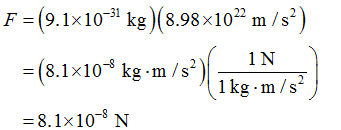Therefore, the force acting on the electron is of magnitude $8.1 \times 10^{-8}$ N, and directed towards the center of the circular orbit.

2. Semiconductor Devices – It’s another very important topic where questions can come from “Logic Gates” in JEE 2018. The question can be as below:

Ques. The combination of? NAND? Gates shown here under (figure) are equivalent to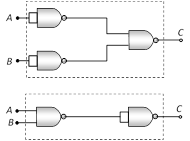1. A) An OR gate and an AND gate respectively
2. B) An AND gate and a NOT gate respectively
3. C) An AND gate and an OR gate respectively
4. D) An OR gate and a NOT gate respectively

Solution:C=A ¯ .B ¯  ¯ ¯ ¯ ¯ ¯ ¯ ¯ ¯ ¯ ¯  =A ¯ +B ¯  ¯ ¯ ¯ ¯ ¯ ¯ ¯ ¯ ¯ ¯ ¯ ¯ ¯ ¯  =A+B

(De Morgan’s theorem) Hence output C is equivalent to OR gate.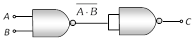C=AB ¯ ¯ ¯ ¯ ¯ ¯ ¯ ¯  .AB ¯ ¯ ¯ ¯ ¯ ¯ ¯ ¯   ¯ ¯ ¯ ¯ ¯ ¯ ¯ ¯ ¯ ¯ ¯ ¯ ¯ ¯ ¯ ¯ ¯  =AB ¯ ¯ ¯ ¯ ¯ ¯ ¯ ¯  +AB ¯ ¯ ¯ ¯ ¯ ¯ ¯ ¯   ¯ ¯ ¯ ¯ ¯ ¯ ¯ ¯ ¯ ¯ ¯ ¯ ¯ ¯ ¯ ¯ ¯ ¯ ¯ ¯ ¯  =AB+AB=AB

In this case output C is equivalent to AND gate.

3. Thermodynamics: The relationship of Cp and Cv is the most common questionnaire in JEE Main exam.

Ques.  A 1-kg block of ice at 0ºC is placed into a perfectly insulated, sealed container that has 2 kg of water also at 0ºC. The water and ice completely fill the container, but the container is flexible. After some time one can accept that

(A) The water will freeze so that the mass of the ice will increase.

(B) The ice will melt so that the mass of the ice will decrease.

(C) Both the amount of water and the amount of ice will remain constant.

(D) Both the amount of water and the amount of ice will decrease

Solution: The correct option is (B) the ice will melt so that the mass of the ice will decrease. In accordance to the second law of thermodynamics, if an irreversible process occurs in a closed system, the entropy of that system always increases; it never decreases. Entropy measures the state of disorder. As the ice is more order than water, therefore the ice will melt so that the mass of the ice will decrease. From the above observation, we conclude that option (B) is correct.

4. Dynamics and Electrostatics – ‘Kirchhoff’s Law’: Another most common question usually you can see on every year on “Kirchhoff’s Law”.

Ques. Calculate the value of current I in the section of networks shown in figure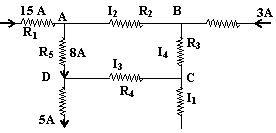Solution: Actually it’s checking the understanding of KCL

At junction A: I2+8 = 15; I2=7A

At junction D: I3 +5 = 8; I3 = 3A

At junction B: I2+3 = I4; I4 =10A

At junction C: I1=I3 + I4; I1 =13 A

5. Magnetic Field: If you follow the last few years question of Physics in JEE Main you can find another common question is from “Magnetic field in a shape”.

Ques. Two straight long conductors AOB and COD are perpendicular to each other and carry currents i1 and i2 .The magnitude of the magnetic induction at a point P at a distance a from the point O in a direction perpendicular to the plane ACBD is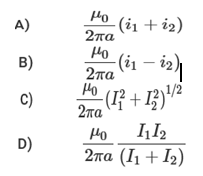Solution: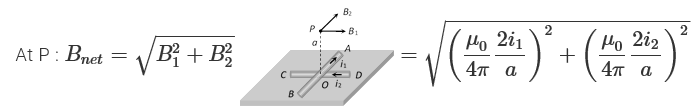Electromagnetic Induction and Alternating Currents: Most common question in this topic usually comes from “Resistor-Inductor-Capacitor”.

Ques. The voltage applied to a pure capacitor of 50*10-6 F is as shown in the figure. Calculate the current for      0-1msec.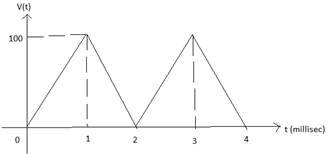1. a) 5A
2. b) 1A
3. c) -5A
4. d) -1A

Solution:    For 0 <= t <= 1msec,
V (t) =mt (y=mx form)
→ 100= 1
10-3m → m= 1105
→  V (t) = 1105t
Current I (t) = c. d (v (t))/dt = 50
10-6* (d (1*105t)/dt) = 5A.

7. Photoelectric effect – Modern Physics: Different types of question on Bohr’s Model and Photoelectric Effect can come in the exam.

Ques. Let K1 be the maximum kinetic energy of photoelectrons emitted by light of wavelength Λand K2 corresponding to wavelength ?2. If λ1= 2 λ2 then,

1. 2K1= K2
2. K1= 2K2
3. K1< K2/2
4. K1> 2K2

Correct Answer: Option (C)

Solution:   As we know, K1 = hc/λ1 – W         …… (1)

and  K2 = hc/λ– W                      …… (2)

Substituting λ1= 2 λ2 in equation (1), we get,

K1 = (hc/2 λ 2) – W = ½ (hc/λ2) – W = 1/2 (K2+W) – W

Thus, K1 = K2/2  – W/2 Or, K1<K2/2

Therefore from the above observation, we conclude that option (C) is correct.